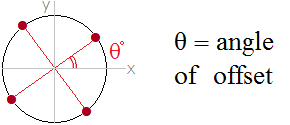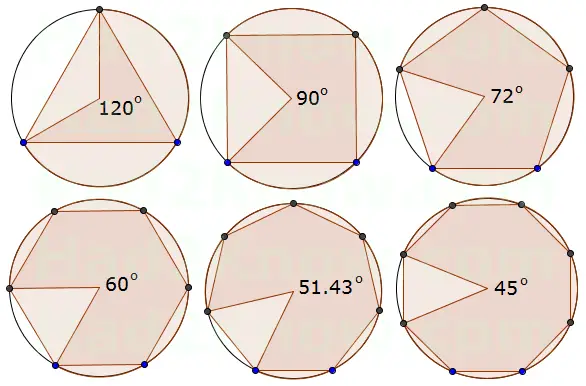# How to Calculate Bolt Positions for a Bolt Circle Pattern

 offset angle degreesdiameter number of bolts 3 4 5 6 7 8

Bolts arranged in a circular or regular polygon pattern offer the most stability and strength for machine parts and wheels. Bolt circle patterns usually have 3 to 8 bolts spaced evenly along the circumference of a circle, forming an equilateral triangle, square, regular pentagon, hexagon, heptagon, or octagon. Bolt circle calculations can be performed using x and y Cartesian coordinates, or angles and diameters.

To calculate bolt placement with Cartesian coordinates, use the bolt pattern calculator at left. Simply plug in the value of the offset angle (if any), the diameter of the circle, and the number of bolts. This calculator assumes that (0,0) is the center of the circle.

To compute the placement of bolts using angles, keep reading below. This article presupposes that you have knowledge of high school geometry. To draw the pattern, you will need a compass, protractor, and a calculator.

## Using Angles to Determine a Bolt Circle Pattern

For a circular bolt pattern with n bolts, the angle between two adjacent bolts is 360/n. The center of the circle is taken as the vertex (point) of the angle.For example, when five bolts are arranged evenly along the outside of a circle, each pair of neighboring bolts is 360/5 = 72 degrees apart, anglewise. The linear distance between two neighboring bolts depends not only on angle, but also on the diameter of the circle. The distance formula for n bolts placed on a circle with a diameter of d is

Distance = d*sin(360/(2n))
= d*sin(180/n)

Be sure to set your calculator to degree mode, rather than radians, when using this formula. Example: if a bolt circle has a diameter of 50 cm and 8 bolts spaced evenly around the circumference, then the distance between two adjacent bolts is

50*sin(180/8)
= 50*sin(22.5)
= 50*(0.38268)
= 19.134 cm

When measuring the distance between bolts, measure from the center of a bolt to the center of another.